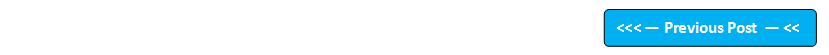Google+

## Blog Pages

### 50. Relational OperatorsProgram to demonstrate the relational operator '==' (i.e.Equal to) class OperatorEqualTo {    public static void main(String args[])    {        int a=3,b=3;        System.out.println(a==b);     //a==b will return 'true' as 3==3 is true    }  } Output of this program: true Program to demonstrate the relational operator '!=' (i.e.Not Equal to) class OperatorNotEqualTo {    public static void main(String args[])    {        int a=3,b=3;        System.out.println(a!=b);      //a!=b will return 'false' as 3!=3 is false    }  } Output of this program: false Program to demonstrate the relational operator '<' (i.e. less than) class OperatorLessThan {    public static void main(String args[])    {        int a=3,b=4,c=6;        System.out.print(" a is less than b is "); System.out.println(a' (i.e. greater than) class OperatorGreaterThan {    public static void main(String args[])    {        int a=3,b=4,c=6;        System.out.print(" a is greater than b is ");        System.out.println(a>b);        System.out.print(" c is greater than b is ");        System.out.println(c>b);    }  } Output of this program: a is greater than b is false c is greater than b is true Program to demonstrate the relational operator '<=' (i.e.less than or Equal to) class OperatorLessThanOrEqualTo {    public static void main(String args[])    {        int a=3,b=3,c=6;        System.out.print(" a is less than or equal to b is ");        System.out.println(a<=b);     //a and b are equal hence a<=b will return true        System.out.print(" a is less than or equal to c is ");        System.out.println(a<=c);    // a is less than c hence a<=c will return true        System.out.print(" c is less than or equal to b is ");        System.out.println(c<=b);   // c is neither less than b nor equal to b hence c<=b will return false    }  } Output of this program: a is less than or equal to b is true a is less than or equal to c is true c is less than or equal to b is false Program to demonstrate the relational operator '>=' (i.e. greater than or Equal to) class OperatorLessThanOrEqualTo {    public static void main(String args[])    {        int a=3,b=3,c=6;        System.out.print(" a is less than or equal to b is ");        System.out.println(a>=b);     //a and b are equal hence a>=b will return true        System.out.print(" a is less than or equal to c is ");        System.out.println(a>=c);    // a is neither greater than c  nor equal to c hence a>=c will return false        System.out.print(" c is less than or equal to b is ");        System.out.println(c>=b);   // c is greater than b  hence c>=b will return true    }  } Output of this program: a is greater than or equal to b is true a is greater than or equal to c is false c is greater than or equal to b is true

Please comment below to feedback or ask questions.

How to use Boolean logical operators will be explained in the next post

#### 1 comment:

suresh ksv said...

Hi Arun, This is Suresh KSV

In S.O.P statement message and output message is different.Please change that in Program to demonstrate the relational operator '>=' (i.e. greater than or Equal to).

System.out.print(" a is less than or equal to b is ");
System.out.println(a>=b); //a and b are equal hence a>=b will return true
System.out.print(" a is less than or equal to c is ");
System.out.println(a>=c); // a is neither greater than c nor equal to c hence a>=c will return false
System.out.print(" c is less than or equal to b is ");
System.out.println(c>=b);

But Output is
a is greater than or equal to b is true
a is greater than or equal to c is false
c is greater than or equal to b is true